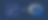# R语言多元线性回归

1. 简介：数据库的目标和简要说明（来源和选择数据库的原因）
2. 规格。所选主题的简要理论发展。变量和数学函数
3. 基础数据处理（即，它将允许回答：是否存在非典型数据？是否存在缺失数据？变量之间是否存在关系？是否需要消除任何变量？）以及对所提出模型的估计和估计参数的解释
4. 模型的验证或确认
5. 预测
6. 脚本

 1 2  Sys.setlocale("LC_TIME", "English") options(stringsAsFactors = FALSE) 

 1 2 3  library(xts) library(ggplot2) library(reshape2) 

 1 2 3  csv_data <- read.csv("./climate_change.csv", header=TRUE, sep=",") head(csv_data, 20) str(csv_data) 
  1 2 3 4 5 6 7 8 9 10 11   Date CO2 CH4 Temp N2O 1 1983-05 345.96 1638.59 0.109 303.677 2 1983-06 345.52 1633.71 0.118 303.746 3 1983-07 344.15 1633.22 0.137 303.795 4 1983-08 342.25 1631.35 0.176 303.839 5 1983-09 340.17 1648.40 0.149 303.901 6 1983-10 340.30 1663.79 0.093 303.970 7 1983-11 341.53 1658.23 0.232 304.032 8 1983-12 343.07 1654.31 0.078 304.082 9 1984-01 344.05 1658.98 0.089 304.130 10 1984-02 344.77 1656.48 0.013 304.194 

 1 2 3 4 5  Date <- csv_data$Date Temp <- csv_data$Temp CH4 <- csv_data$CH4 CO2 <- csv_data$CO2 N2O <- csv_data$N2O  设置日期为索引和没有日期的 datafram，并查看最大值、最小值、方差等等  1 2 3 4 5  format_date <- as.Date(paste0(as.character(csv_data[,1]), "-01"), format='%Y-%m-%d') data <- xts(csv_data[,-1], order.by = format_date) head(data, 10) mdata <- data.frame(Temp, CH4, CO2, N2O) summary(mdata)    1 2 3 4 5 6 7 8 9 10 11   CO2 CH4 Temp N2O 1983-05-01 345.96 1638.59 0.109 303.677 1983-06-01 345.52 1633.71 0.118 303.746 1983-07-01 344.15 1633.22 0.137 303.795 1983-08-01 342.25 1631.35 0.176 303.839 1983-09-01 340.17 1648.40 0.149 303.901 1983-10-01 340.30 1663.79 0.093 303.970 1983-11-01 341.53 1658.23 0.232 304.032 1983-12-01 343.07 1654.31 0.078 304.082 1984-01-01 344.05 1658.98 0.089 304.130 1984-02-01 344.77 1656.48 0.013 304.194   1 2 3 4 5 6 7   Temp CH4 CO2 N2O Min. :-0.2820 Min. :1630 Min. :340.2 Min. :303.7 1st Qu.: 0.1217 1st Qu.:1722 1st Qu.:353.0 1st Qu.:308.1 Median : 0.2480 Median :1764 Median :361.7 Median :311.5 Mean : 0.2568 Mean :1750 Mean :363.2 Mean :312.4 3rd Qu.: 0.4073 3rd Qu.:1787 3rd Qu.:373.5 3rd Qu.:317.0 Max. : 0.7390 Max. :1814 Max. :388.5 Max. :322.2  创建每个变量的时间序列，这里的 png()dev.off() 用于将生成的图片保存到本地，你也可以删除它直接在 R 语言程序中预览 由于作业需要，我创建了四张图片，你也可以将四张表格放在一张图片上。   1 2 3 4 5 6 7 8 9 10 11 12 13 14 15 16 17 18 19 20 21 22 23 24 25 26 27  # 温度时间序列 png(file = "./images/time_series_Temp.png", width = 800, height = 525, res = 100) par(oma=c(2,2,2,2)) time_series_Temp <- xts(Temp, order.by = format_date) (time_series_Temp, main="Series temporales de temperatura", xlab="Date", ylab="Temp") dev.off() # CO2时间序列 png(file = "./images/time_series_CO2.png", width = 800, height = 525, res = 100) par(oma=c(2,2,2,2)) time_series_CO2 <- xts(CO2, order.by = format_date) plot(time_series_CO2, main="Series temporales de CO2", xlab="Date", ylab="CO2") dev.off() # CH4时间序列 png(file = "./images/time_series_CH4.png", width = 800, height = 525, res = 100) par(oma=c(2,2,2,2)) time_series_CH4 <- xts(CH4, order.by = format_date) plot(time_series_CH4, main="Series temporales de CH4", xlab="Date", ylab="CH4") dev.off() # N2O时间序列 png(file = "./images/time_series_N2O.png", width = 800, height = 525, res = 100) par(oma=c(2,2,2,2)) time_series_N2O <- xts(N2O, order.by = format_date) plot(time_series_N2O, main="Series temporales de N2O", xlab="Date", ylab="N2O") dev.off()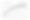time_series_CH4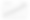time_series_CO2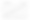time_series_N2O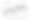time_series_Temp 创建所有变量之间的关系图  1 2 3 4  png(file = "./images/correlation_all.png", width = 800, height = 525, res = 100) par(oma=c(2,2,2,2)) plot(mdata) dev.off()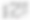correlation_all 创建每个变量之间的散点图   1 2 3 4 5 6 7 8 9 10 11 12 13 14 15 16 17 18 19 20 21 22 23 24 25 26 27 28 29 30 31 32  # 散点图 温度-N2O png(file = "./images/scatter_Temp_N2O.png", width = 600, height = 525, res = 100) par(oma=c(1,1,1,1)) model_Temp_y_N2O <- lm(Temp~N2O, data=mdata) coef <- coef(model_Temp_y_N2O) eq = paste('y =', round(coef(model_Temp_y_N2O)[], 3), 'x', '+', round(coef(model_Temp_y_N2O)[], 3)) plot(N2O, Temp, xlab="N2O", ylab="Temperatura", pch=20, main="N2O y Temperatura") abline(model_Temp_y_N2O, col="red") mtext(eq, side = 3, line=1, adj = 0, padj = 1, col="blue") dev.off() # 散点图 温度-CH4 png(file = "./images/scatter_Temp_CH4.png", width = 600, height = 525, res = 100) par(oma=c(1,1,1,1)) model_Temp_y_CH4 <- lm(Temp~CH4, data=mdata) coef <- coef(model_Temp_y_CH4) eq = paste('y =', round(coef(model_Temp_y_CH4)[], 3), 'x', '+', round(coef(model_Temp_y_CH4)[], 3)) plot(CH4, Temp, xlab="CH4", ylab="Temperatura", pch=20, main="CH4 y Temperatura") abline(model_Temp_y_CH4, col="red") mtext(eq, side = 3, line=1, adj = 0, padj = 1, col="blue") dev.off() # 散点图 温度-CO2 png(file = "./images/scatter_Temp_CO2.png", width = 600, height = 525, res = 100) par(oma=c(1,1,1,1)) model_Temp_y_CO2 <- lm(Temp~CO2, data=mdata) coef <- coef(model_Temp_y_CO2) eq = paste('y =', round(coef(model_Temp_y_CO2)[], 3), 'x', '+', round(coef(model_Temp_y_CO2)[], 3)) plot(CO2, Temp, xlab="CO2", ylab="Temperatura", pch=20, main="CO2 y Temperatura") abline(model_Temp_y_CO2, col="red") mtext(eq, side = 3, line=1, adj = 0, padj = 1, col="blue") dev.off()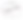scatter_Temp_N2O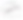scatter_Temp_CO2scatter_Temp_N2O 创建直方图   1 2 3 4 5 6 7 8 9 10 11 12 13 14 15 16 17 18 19 20 21 22 23  # 因变量(温度)直方图 png(file = "./images/hist_Temp.png", width = 800, height = 525, res = 100) par(oma=c(2,2,2,2)) hist(Temp, breaks=100, col="pink", main="Histograma de Temperatura", xlab="Temperatura", ylab="Frecuencia") dev.off() # 自变量(CH4)直方图 png(file = "./images/hist_CH4.png", width = 800, height = 525, res = 100) par(oma=c(2,2,2,2)) hist(CH4, breaks=100, col="pink", main="Histograma de CH4", xlab="CH4", ylab="Frecuencia") dev.off() # 自变量(CO2)直方图 png(file = "./images/hist_CO2.png", width = 800, height = 525, res = 100) par(oma=c(2,2,2,2)) hist(CO2, breaks=100, col="pink", main="Histograma de CO2", xlab="CO2", ylab="Frecuencia") dev.off() # 自变量(N2O)直方图 png(file = "./images/hist_N2O.png", width = 800, height = 525, res = 100) par(oma=c(2,2,2,2)) hist(N2O, breaks=100, col="pink", main="Histograma de N2O", xlab="N2O", ylab="Frecuencia") dev.off()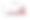hist_CH4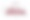hist_CO2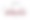hist_N2Ohist_N2O 创建箱线图来检测异常值   1 2 3 4 5 6 7 8 9 10 11 12 13 14 15 16 17 18 19 20 21 22 23  # 检测异常值(Temp) png(file = "./images/boxplot_Temp.png", width = 800, height = 525, res = 100) par(oma=c(2,2,2,2)) boxplot(CO2, main="Boxplot de CO2", xlab="CO2", ylab="Frecuencia") dev.off() # 检测异常值(CH4) png(file = "./images/boxplot_CH4.png", width = 800, height = 525, res = 100) par(oma=c(2,2,2,2)) boxplot(CH4, main="Boxplot de CH4", xlab="CH4", ylab="Frecuencia") dev.off() # 检测异常值(CO2) png(file = "./images/boxplot_N2O.png", width = 800, height = 525, res = 100) par(oma=c(2,2,2,2)) boxplot(N2O, main="Boxplot de N2O", xlab="N2O", ylab="Frecuencia") dev.off() # 检测异常值(CO2) png(file = "./images/boxplot_CO2.png", width = 800, height = 525, res = 100) par(oma=c(2,2,2,2)) boxplot(Temp, main="Boxplot de Temperatura", xlab="Temperatura", ylab="Frecuencia") dev.off()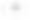boxplot_CH4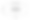boxplot_CO2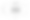boxplot_N2O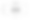boxplot_Temp 查看相关系数  1 2  # 相关系数 cor(mdata)   1 2 3 4 5   Temp CH4 CO2 N2O Temp 1.0000000 0.6996966 0.7485046 0.7432418 CH4 0.6996966 1.0000000 0.8722531 0.8944092 CO2 0.7485046 0.8722531 1.0000000 0.9811354 N2O 0.7432418 0.8944092 0.9811354 1.0000000  建立线性模型，并打印参数估计的结果，然后进行回归方程的显著性检验 • T检验 • F检验 • 调整后的 Adjusted R²  1 2 3 4  # 建立线形模型 modelo <- lm(formula=Temp~CO2+CH4+N2O,data=data) modelo summary(modelo)  最后，我们通过的回归参数的检验与回归方程的检验，得到最后多元线性回归方程为： Temp = -4.2676862 + 0.0076848 * CO2 + 0.0007349 * CH4 + 0.0014314 * N2O   1 2 3 4 5 6 7 8 9 10 11 12 13 14 15 16 17 18 19  Call: lm(formula = Temp ~ CO2 + CH4 + N2O, data = data) Residuals: Min 1Q Median 3Q Max -0.41044 -0.07476 0.00139 0.07208 0.44757 Coefficients: Estimate Std. Error t value Pr(>|t|) (Intercept) -4.2676862 1.1577046 -3.686 0.000269 *** CO2 0.0076848 0.0027617 2.783 0.005729 ** CH4 0.0007349 0.0003278 2.242 0.025699 * N2O 0.0014314 0.0073093 0.196 0.844868 --- Signif. codes: 0 '***' 0.001 '**' 0.01 '*' 0.05 '.' 0.1 ' ' 1 Residual standard error: 0.1181 on 304 degrees of freedom Multiple R-squared: 0.5695, Adjusted R-squared: 0.5652 F-statistic: 134 on 3 and 304 DF, p-value: < 2.2e-16  在得到的回归模型进行显著性检验后，还要在做残差分析(预测值和实际值之间的差)，检验模型的正确性，残差必须服从正态分布N(0,σ^2)。直接用plot()函数生成4种用于模型诊断的图形，进行直观地分析。  1 2 3 4  png(file = "./images/residual_analysis.png", width = 1000, height = 625, res = 90) par(mfrow=c(2,2)) plot(modelo) dev.off()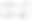residual_analysis 逐步回归分析  1  step(modelo)    1 2 3 4 5 6 7 8 9 10 11 12 13 14 15 16 17 18 19 20 21 22 23  Start: AIC=-1312.01 Temp ~ CO2 + CH4 + N2O Df Sum of Sq RSS AIC - N2O 1 0.000535 4.2397 -1314.0 4.2391 -1312.0 - CH4 1 0.070077 4.3092 -1309.0 - CO2 1 0.107975 4.3471 -1306.3 Step: AIC=-1313.97 Temp ~ CO2 + CH4 Df Sum of Sq RSS AIC 4.2397 -1314.0 - CH4 1 0.09021 4.3299 -1309.5 - CO2 1 0.78620 5.0259 -1263.6 Call: lm(formula = Temp ~ CO2 + CH4, data = data) Coefficients: (Intercept) CO2 CH4 -4.0469071 0.0081818 0.0007611  计算 R²   1 2 3 4 5 6 7 8 9 10 11  # 计算去除 CO2 后的R² modelo_del_CO2 <- lm(formula=Temp~CH4+N2O,data=mdata) summary(modelo_del_CO2) # 计算去除 CH4 后的R² modelo_del_CH4 <- lm(formula=Temp~CO2+N2O,data=mdata) summary(modelo_del_CH4) # 计算去除 N2O 后的R² modelo_del_N2O <- lm(formula=Temp~CO2+CH4,data=mdata) summary(modelo_del_N2O)    1 2 3 4 5 6 7 8 9 10 11 12 13 14 15 16 17 18 19 20 21 22 23 24 25 26 27 28 29 30 31 32 33 34 35 36 37 38 39 40 41 42 43 44 45 46 47 48 49 50 51 52 53 54 55 56 57 58 59 60  Call: lm(formula = Temp ~ CH4 + N2O, data = mdata) Residuals: Min 1Q Median 3Q Max -0.40339 -0.06894 -0.00508 0.07248 0.44869 Coefficients: Estimate Std. Error t value Pr(>|t|) (Intercept) -7.2171582 0.4707278 -15.332 <2e-16 *** CH4 0.0006792 0.0003308 2.053 0.0409 * N2O 0.0201206 0.0029156 6.901 3e-11 *** --- Signif. codes: 0 '***' 0.001 '**' 0.01 '*' 0.05 '.' 0.1 ' ' 1 Residual standard error: 0.1194 on 305 degrees of freedom Multiple R-squared: 0.5585, Adjusted R-squared: 0.5556 F-statistic: 192.9 on 2 and 305 DF, p-value: < 2.2e-16 Call: lm(formula = Temp ~ CO2 + N2O, data = mdata) Residuals: Min 1Q Median 3Q Max -0.41767 -0.07982 -0.00245 0.06403 0.45301 Coefficients: Estimate Std. Error t value Pr(>|t|) (Intercept) -4.934714 1.126173 -4.382 1.62e-05 *** CO2 0.007307 0.002775 2.633 0.00889 ** N2O 0.008123 0.006716 1.210 0.22740 --- Signif. codes: 0 '***' 0.001 '**' 0.01 '*' 0.05 '.' 0.1 ' ' 1 Residual standard error: 0.1189 on 305 degrees of freedom Multiple R-squared: 0.5624, Adjusted R-squared: 0.5595 F-statistic: 196 on 2 and 305 DF, p-value: < 2.2e-16 Call: lm(formula = Temp ~ CO2 + CH4, data = mdata) Residuals: Min 1Q Median 3Q Max -0.41026 -0.07505 0.00251 0.07367 0.44738 Coefficients: Estimate Std. Error t value Pr(>|t|) (Intercept) -4.0469071 0.2629079 -15.393 < 2e-16 *** CO2 0.0081818 0.0010879 7.521 6.14e-13 *** CH4 0.0007611 0.0002988 2.548 0.0113 * --- Signif. codes: 0 '***' 0.001 '**' 0.01 '*' 0.05 '.' 0.1 ' ' 1 Residual standard error: 0.1179 on 305 degrees of freedom Multiple R-squared: 0.5694, Adjusted R-squared: 0.5666 F-statistic: 201.7 on 2 and 305 DF, p-value: < 2.2e-16  进行预测  1 2 3 4 5  nuevos_datos <- data.frame(CH4=1700,CO2=350) nuevos_datos pred <- predict(object=modelo_del_N2O, data=nuevos_datos, interval="prediction",level=0.95) pred head(pred,10)    1 2 3 4 5 6 7 8 9 10 11   fit lwr upr 1 0.030839372 -2.045871e-01 0.2662658 2 0.023525076 -2.122342e-01 0.2592844 3 0.011943094 -2.236052e-01 0.2474914 4 -0.005025586 -2.404213e-01 0.2303702 5 -0.009066399 -2.432822e-01 0.2251494 6 0.003711027 -2.300327e-01 0.2374547 7 0.009542722 -2.243235e-01 0.2434090 8 0.019159020 -2.149089e-01 0.2532269 9 0.030731635 -2.031753e-01 0.2646386 10 0.034719685 -1.993524e-01 0.2687918  我们得到了多元线性回归方程的公式，就可以对数据进行预测了。我们可以用R语言的predict()函数来计算预测值y0和相应的预测区间，并把实际值和预测值一起可视化化展示。   1 2 3 4 5 6 7 8 9 10 11 12 13 14 15 16 17 18 19 20 21 22  png(file = "./images/prediction.png", width = 1000, height = 625, res = 90) par(mfrow=c(1,1)) dfp <- data.frame(pred) head(dfp, 10) mode(dfp) mdf<-merge(data$Temp, fit=dfp$fit, lwr=dfp$lwr, upr=dfp$upr) mdf # 画图函数 draw <- function(df){ df2<-data.frame(df) df2$id<-index(df2) df2\$date<-index(df) df3<-melt(df2,id=c("id","date")) g<-ggplot(data=df3,aes_string(x='id',y='value',colour='variable')) g<-g+geom_line() g } draw(mdf) dev.off() 
  1 2 3 4 5 6 7 8 9 10 11 12 13 14 15 16 17 18 19 20 21 22 23 24   Temp fit lwr upr 1983-05-01 0.109 0.030839372 -2.045871e-01 0.2662658 1983-06-01 0.118 0.023525076 -2.122342e-01 0.2592844 1983-07-01 0.137 0.011943094 -2.236052e-01 0.2474914 1983-08-01 0.176 -0.005025586 -2.404213e-01 0.2303702 1983-09-01 0.149 -0.009066399 -2.432822e-01 0.2251494 1983-10-01 0.093 0.003711027 -2.300327e-01 0.2374547 1983-11-01 0.232 0.009542722 -2.243235e-01 0.2434090 1983-12-01 0.078 0.019159020 -2.149089e-01 0.2532269 1984-01-01 0.089 0.030731635 -2.031753e-01 0.2646386 1984-02-01 0.013 0.034719685 -1.993524e-01 0.2687918 1984-03-01 0.049 0.039824705 -1.943438e-01 0.2739932 1984-04-01 -0.019 0.051996586 -1.821958e-01 0.2861889 1984-05-01 0.065 0.052022929 -1.828336e-01 0.2868794 1984-06-01 -0.016 0.035790138 -2.002370e-01 0.2718173 1984-07-01 -0.024 0.020863011 -2.152485e-01 0.2569745 1984-08-01 0.034 0.012124223 -2.225349e-01 0.2467834 1984-09-01 0.025 0.012157273 -2.215542e-01 0.2458687 1984-10-01 -0.035 0.023267748 -2.102364e-01 0.2567719 1984-11-01 -0.123 0.036423363 -1.969677e-01 0.2698144 1984-12-01 -0.282 0.044764790 -1.886841e-01 0.2782137 1985-01-01 -0.001 0.043929180 -1.898457e-01 0.2777041 1985-02-01 -0.155 0.052679969 -1.810188e-01 0.2863788 1985-03-01 -0.032 0.075003316 -1.583058e-01 0.3083124 

El trabajo está bastante bien. Os sugiero para otros trabajos que: numeréis las páginas, pongáis título y número a las tablas y gráficas, mejorar redacción y ser más explícitos en la redacción. (por favor revisad comentarios dentro del texto). Puntuación según apartados:

Introducción (0.25/1)

Especificación (1/1)

Tratamiento, estimación e inter. (2.5/3)

Verificación (2/2.5)

Predicción (1/1)

Script(1.5/1.5)